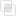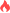python有哪些数组叠加函数

Python 数组 函数2021-09-10 09:56:171121浏览 · 0收藏 · 0评论1、hstack代表水平方向叠加，要想叠加成功，行必须一致。

2、vstack代表垂直方向叠加。如果叠加成功，列必须一致。

3、concatenate手动指定叠加方向。

axis=0表示垂直方向叠加，axis=1表示水平方向叠加，axis=None表示一维数组叠加。

```import numpy as np
h1 = np.random.randint(0,10,size=(3,1))
h1 #结果:
'''array([,
,
])'''

h2 = np.random.randint(0,10,size=(3,4))
h2 #结果：
'''array([[6, 9, 5, 0],
[6, 1, 9, 4],
[8, 8, 9, 8]])'''
h4 = np.random.randint(0,10,size=(1,4))
h4 # 结果
'''array([[2, 3, 5, 5]])'''

# 2.横向堆叠
h3 = np.hstack([h1,h2])
h3 #结果：
'''array([[4, 6, 9, 5, 0],
[8, 6, 1, 9, 4],
[2, 8, 8, 9, 8]])'''

# 3.使用concatenate进行自定义拼接
np.concatenate([h1,h2],axis=1) #横向拼接 结果：
'''array([[4, 6, 9, 5, 0],
[8, 6, 1, 9, 4],
[2, 8, 8, 9, 8]])'''

# 3.使用concatenate进行自定义堆叠
np.concatenate([h1,h2],axis=None)#拼接成一维数组 结果：
'''
array([4, 8, 2, 6, 9, 5, 0, 6, 1, 9, 4, 8, 8, 9, 8])
'''
# 3.使用concatenate进行自定义堆叠
np.concatenate([h2,h3],axis=0)#纵向拼接 结果
'''array([[6, 9, 5, 0],
[6, 1, 9, 4],
[8, 8, 9, 8],
[2, 3, 5, 5]])'''```python插入排序的优化python插入排序的性能问题python希尔排序的用法python中Git分支操作python中Git的常用命令python中分支管理策略的实现python中分支管理的注意点python魔法函数在类中的使用python公有成员和私有成员的介绍python调用父类的三种方法python继承的缺点python中MRO原则的使用python命令行模式的使用流程python算术运算符的扩展功能python不同类型的对象特点php多条件查询数据库的实现方法869

php去掉尖括号的方法1402

php查询某个字段的方法1381